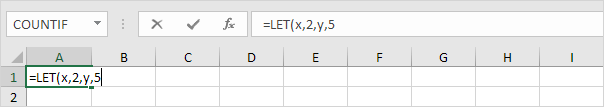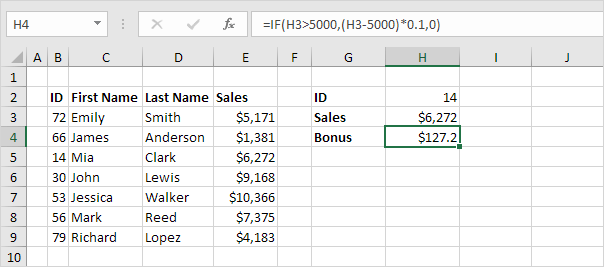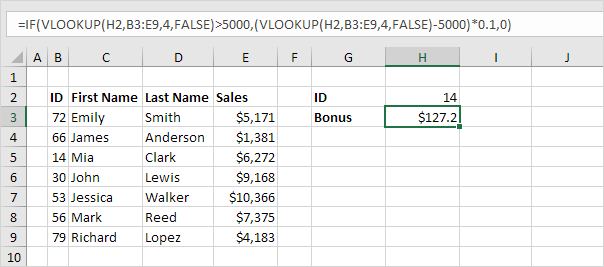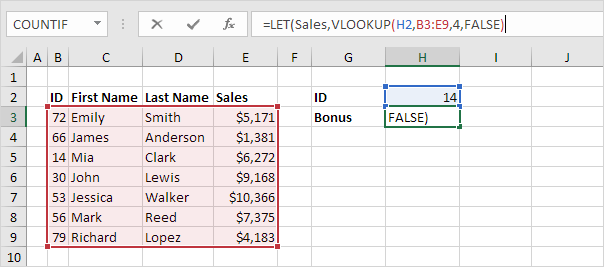# LET function

Use the LET function in Excel 365/2021 to declare variables and assign values (or calculation results) to variables. Next, use these variables in a calculation.

### Simple LET function

1. The simple LET function below declares variable x (and assigns the value 2) and declares variable y (and assigns the value 5).Note: the LET function can handle up to 126 variable/value pairs.

2. The final argument of the LET function is always a calculation. In this example, x+y reduces to 2+5=7.Why using the LET function in Excel? The LET function can make your formulas easier to read. Let's take a look at a cool example.

1. The VLOOKUP function below returns the sales of ID 14.2. The formula below calculates a bonus (10 percent of every dollar above \$5,000).3. To write a single formula, simply replace H3 with the VLOOKUP function.This IF formula is quite difficult to read. Simply use the LET function to make it easier to read.

4. The LET function below declares the variable Sales and assigns a calculation result!5. Next, use the variable Sales in a final calculation.Note: the IF formula is now easier to understand. This LET function has only 1 variable/value pair.

Go to Next Chapter: Sort